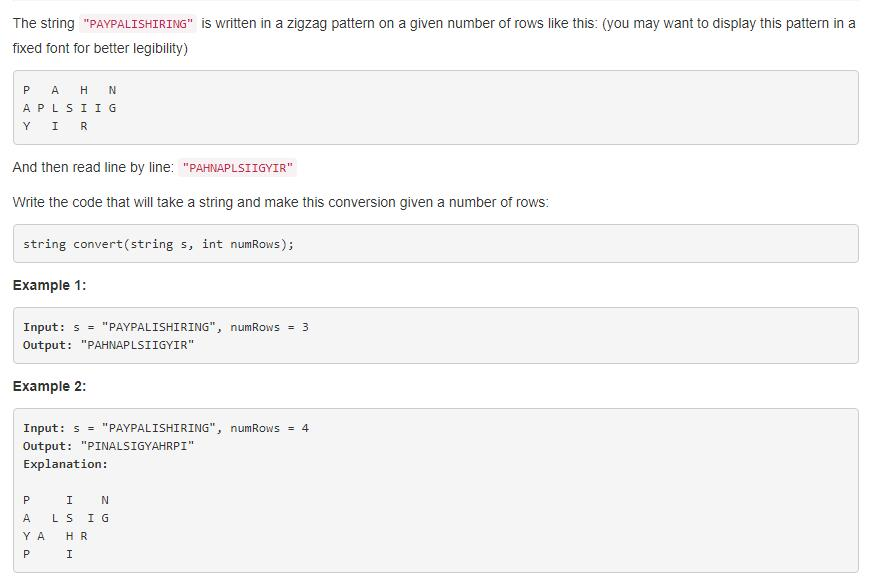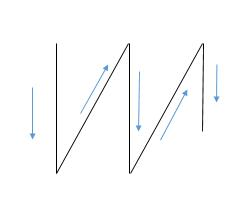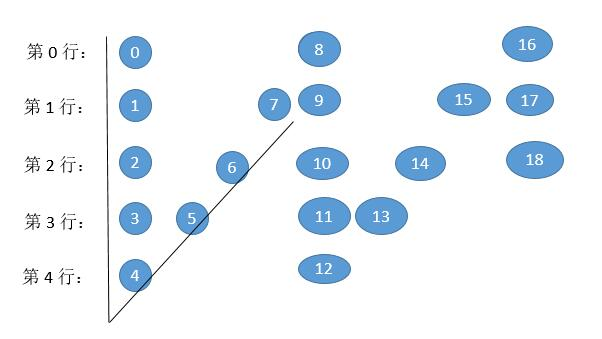## 1. 题目描述（中等难度）## 2. 解法一

 public String convert(String s, int numRows) {

if (numRows == 1) return s;

List<StringBuilder> rows = new ArrayList<>();
for (int i = 0; i < Math.min(numRows, s.length()); i++)

int curRow = 0;
boolean goingDown = false;

for (char c : s.toCharArray()) {
rows.get(curRow).append(c);
if (curRow == 0 || curRow == numRows - 1) goingDown = !goingDown; //遍历到两端，改变方向
curRow += goingDown ? 1 : -1;
}

StringBuilder ret = new StringBuilder();
for (StringBuilder row : rows) ret.append(row);
return ret.toString();
}


## 3. 解法二public String convert(String s, int numRows) {

if (numRows == 1)
return s;

StringBuilder ret = new StringBuilder();
int n = s.length();
int cycleLen = 2 * numRows - 2;

for (int i = 0; i < numRows; i++) {
for (int j = 0; j + i < n; j += cycleLen) { //每次加一个周期
ret.append(s.charAt(j + i));
if (i != 0 && i != numRows - 1 && j + cycleLen - i < n) //除去第 0 行和最后一行
ret.append(s.charAt(j + cycleLen - i));
}
}
return ret.toString();
}